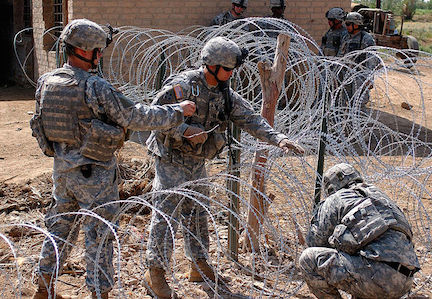# The Stingy Rancher And His Fence

Calculus Level 4A rancher has been given a square mile of land, a perfect square with a perimeter of 4 miles. He decides he is going to fence in his property, but fencing costs money, and so he's only interested in fencing in the most land he can for the cost of the fencing, which is \$1000 per mile. Let $A$ be the area of the land fenced in, and $B$ denote the total cost of the fence. When $A/B$ is maximized, how much did he pay for fencing, expressed as $\left\lfloor B \right\rfloor ?$

Details and Assumptions

• There is no existing fencing on the land given to the rancher, including at the boundary.
• Because the area is bounded, $\frac{A}{B}$ will be bounded above. For example, we are not allowed to use a circle of radius 1.

This problem was suggested by Open Question...

×